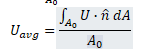# How to access Spreadsheet data

Hello everyone!

I have used OpenFOAM for a CFD simulation and right now I am manually trying to obtain the data I need. One of those is the Area averaged Velocity:In order:

• I have used the slice tool to get the area of interest
• Used the “generate Surface normal” filter
• Calculated with the calculator filter the quantity abs(U.Normals)
• Implemented an integration by means of “IntegrateVariables”, activating the “divide per cell area/volume” function

I can correctly compute the area averaged velocity, but I need to isolate the value from the spreadsheet row in order to use this value in successive operations.

How can I do that?

thanks in advance for your helpDo you want to use the value as a property value in a filter? That’s not easy, I’m afraid.

Or do you want to use it in a subsequent filtering operation? You can use the Programmable Filter to access the value in the table.

Hi and thank you for your very fast reply! I need it in order to calculate a flow uniformity index. I used the Catalyst script generator. Ideally, I would like to select a 2D Surface (a slice) and to obtain a flow uniformity index. The script generated by catalyst is the following:

# create a new ‘Generate Surface Normals’

generateSurfaceNormals = GenerateSurfaceNormals(Input=slice)

# create a new ‘Calculator’

phiCalc = Calculator(Input=generateSurfaceNormals)
phiCalc.ResultArrayName = ‘phiCalc’
phiCalc.Function = 'abs(mag(U)-UavgAveraged

# create a new ‘Calculator’

uavg = Calculator(Input=generateSurfaceNormals)
uavg.ResultArrayName = ‘Uavg’
uavg.Function = ‘abs(U.Normals)’

# create a new ‘Point Data to Cell Data’

pointDatatoCellData1 = PointDatatoCellData(Input=uavg)

# create a new ‘Integrate Variables’

integrateVariables1 = IntegrateVariables(Input=pointDatatoCellData1)
integrateVariables1.DivideCellDataByVolume = 1

# create a new ‘Point Data to Cell Data’

pointDatatoCellData2 = PointDatatoCellData(Input=phiCalc)

# create a new ‘Integrate Variables’

integrateVariables2 = IntegrateVariables(Input=pointDatatoCellData2)

From the final integration the area averaged phiCalc (let’s say phiCalcAveraged) should be collected, in order to compute:

UI=1-phiCalcAveraged/(2*UavgAveraged)

How can I implement it?Sorry for the delay in response.

You’ll need to `Fetch` the results of the integrate variables filter from the server proxy layer and use them in the expression. It’ll look like this:

``````from vtkmodules.numpy_interface import dataset_adapter as dsa
local_integrateVariables1 = dsa.WrapDataObject(servermanager.Fetch(integrateVariables1))
UavgAveraged = local_integrateVariables1.CellData['Uavg']
``````

You’ll do the same for the second Integrate Variables filter.

Alternate method:-

Can we Fetch the values from dsa